## Saturday, February 26, 2011

### Alec's Winnipeg Harvest Scribe Post

I learned that brown rice is healthier than white rice.I also learned that some people who volunteered in Winnipeg Harvest also experienced of not having food and been help by Winnipeg Harvest. They want to return the favor by working in Winnipeg Harvest whenever they had a free time . Winnipeg Harvest helped people by giving them food to eat. Stores liked Superstore and Costco helps Winnipeg harvest to supply food by giving them canned goods, milk and juiced.The top ten items that is needed in Winnipeg Harvest are:
1. Canned fish and poultry.
2. Canned fruit and vegetables (packed in own juiced)
3. Canned stew, chili, brown beans
4. Peanut Butter (light)
5. Baby Food
6. Whole grain pasta/ Whole wheat pasta
7. Rice -Brown, Converted, Parboiled
8. Canned Spaghetti or Tomatoes
9. Cereal - High fiber, non-sugar coated
10. Canned soup - Lentil, Pea, Vegetable
When I volunteered I was the one who scoop the rice and put it in a plastic.

### Kevin's Winnipeg Harvest Scribe Post

What I learned from Winnipeg Harvest was that 823,856 people use food banks each month. Another thing I learned was that Manitoba has the one of the highest percentage of child food bank clients in Canada 46.6%! Winnipeg Harvest helps people that really can't find food to feed themselves or can't find the food to feed there children. Winnipeg Harvest gets there food from supermarkets such as Superstore, and others from the kind people that come to donate food. The top 10 items are as listed
1. Canned fish and poultry.
2. Canned fruit and vegetables
3. Canned stew, chili, brown beans
4. Peanut Butter (light)
5. Baby Food
6. Whole grain pasta/ Whole wheat pasta
7. Rice (Brown, Converted, Parboiled)
8. Canned Spaghetti or Tomatoes
9. Cereal - High fiber, non-sugar coated
10. Canned soup - Lentil, Pea, Vegetable
When I volunteered I remember i was the rice carrier as in I get the rice from the boxes and open them. Then I pour the rice into the containers for my group to package. It went from white rice to brown rice to parboiled/converted. We also noticed the the parboiled was lighter then white rice or brown rice.

## Sunday, February 20, 2011

### Ryan's Volume Scribe Post

The questions I answered were numbers 4, 6, 8 in the homework book (pg. 80-81).
4. Calculate the volume of each rectangular prism.

a) l = 15 cm, w = 12 cm, h = 3 cm
v = l x w x h
v = 15 x 12 x 3
v = 180 x 3
v = 540cm3Jumbo
r = d/2
r = 20 / 2
r = 10cm

v = (πr2) x h
v = (3.14 x 102) x 40
v = (3.14 x 100) x 40
v = 314 x 40
v = 12, 560cm3

Popcorn Lover's
r = d/2
r = 30/2
r = 15cm

v = (πr^2) x h
v = (3.14 x 15^2) x 20
v = (3.14 x 225) x 20
v = 706.5 x 20
v = 14, 130cm^3

Popcorn Lover's has more space.Full Circle
r = d/2
r = 1/2
r = 0.5m

v = (πr^2) x h
v = (3.14 x 0.5^2) x 10
v = (3.14 x 0.25) x 10
v = 0.785 x 10
v = 7.9m^3

"Hole" Circle
r = d/2
r = 0.8/2
r = 0.4m

v = (πr^2) x h
v = (3.14 x 0.4^2) x 10
v = (3.14 x 0.16) x 10
v = 0.5024 x 10
v = 5.1m^3

7.9 - 5.1 = 2.8m^3

Concrete volume is 2.8m^3.

## Thursday, February 17, 2011

### Melanie's Volume Scribe Post

For my post, I will be answering the questions 4, 5, and 6 on the textbook work we did in class today. ( pages 258 - 261 )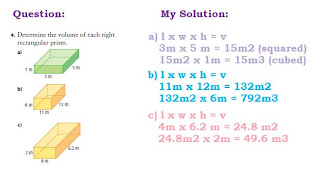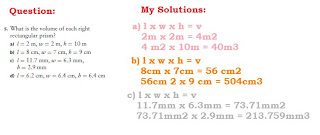*Sorry if some of the pictures are hard to see, just click it and it will appear bigger.

*Here is a link that will hopefully help those of you who don't understand how to find the volume of a rectangle / square.

If you guys don't

Cylinder Volume and Volume Problemsv= π x r x r
v= 3.14 x 12cm x 12 cm
v= 452.16 cm2 x 15
v= 6782.4 cm3

6782.4 cm3 / 4= 1695.6 cm3

The volume of cheese taken from the block was 1695.6 cm3.
One assumption I made was that they cut 1/4 from the cheese.r= d/2
r= 0.8m/2
r= 0.4 m

v=
π x r x r
v= 3.14 x 4cm x 4cm
v= 0.5024 cm2 x 10cm
v= 5.024 m3

r= d/2
r= 1m/2
r= 0.5m

v= π x r x r
v= 3.14 x 0.5m x 0.5m
v= 0.785 m2 x 10m
v= 7.85 m3

7.85 m3 - 5.024 m3 = 2.826 m3

The volume of concrete required to make the culvert is 2.826 m3.

Here is my volume video:

## Wednesday, February 16, 2011

### Kristel's Volume Scribe Post

3. Determine the volume of each right prism or cylinder.

a) v= area of base x height
15 cm2 x 4 cm
60 cm3

b) v= area of base x height
18 cm2 x 12 cm
216 cm3

c) v= area of base x height
96 cm2 x 20 cm
1920 cm3

9. How many ways can you build a rectangular prism from 16 centimeter cubes? Use diagrams or centimeter cubes to show your designs.

You can build 4 designs:

1 - L = 16 cm, W = 1 cm, H = 1 cm

2 - L = 4 cm, W = 2 cm, H = 2 cm

3 - L = 8 cm, W = 1 cm, H = 2 cm

4 - L = 4 cm, W = 1 cm, H = 4 cm

11. José is having vegetable soup. The area of the base of the soup can is 10.4 cm2, and the height is 10 cm. When José opens the can, he sees that the soup comes up to a height of only 9 cm. What volume of soup is in the can?

v = Area of base x height
10. 4 cm2 x 9 cm
93.60 cm3

15. The International Space Station is shaped like a cylinder that has a cross-sectional area of 615 m2 and a length of 44.5 m. The living space for the astronauts is 425 m3. What percent of the volume of the space station is used for living?

Cylinder volume and volume problems

12) 7.3- Page 266Jumbo

d/2 = r

20/2 = r
10 cm = r

v = π x r x r x h
v = (3.14 x 10 x 10) x 40
v = 12560 cm^

Popcorn Lovers
d/2 = r
30/2 = r
15 cm = r

v = π x r x r x h
v = (3.14 x 15 x 15) x 20
v = 14130 cm^

Martha should buy Popcorn Lovers because the volume is larger than the Jumbo

5) 7.4- Page 273d/2 = r
1/2 = r
0.5 m = r

v = π x r x r x h
v = (3.14 x 0.5 x 0.5) x 10
v = 7.850 m^

d/2 = r
0.8/2 = r
0.4 m = r

v = π x r x r x h
v = (3.14 x 0.4 x 0.4) x 10
v = 5.024 m^

7.850 - 5.024 = 2.286 m^

You will need 2.286m^ of concrete

### Jocelle's Scribe PostShow you know: a) What is the volume of the right rectangular prism?

8.5 cm x 4cm = 34 cm²
34 cm² x 5 cm = 170 cm³b) What is the volume of a cube with edge length 3 meters?

Answer: Length x Width x Height
3 cm x 3 cm = 9 cm²
9 cm² x 3 cm = 27 cm ³What is the volume of the right triangular prism?

Answer: base x height ÷ 2 x height
5 x 9 ÷ 2 = 22.5 mm²
22.5 mm² x 8 mm = 180 mm³

My Cylinder Volume and Volume Problemr = d/2
r = 20/2
r = 10 cm²

v = π x r x r x h
( 3.14 x 10 x 10 ) x 40
314 x 40
1,2560 cm³

r = d/2
r = 30/2
r = 15 cm
²

v = π x r x r x h
( 3.14 x 15 x 15 ) x 40
14, 130 cm
³

## Tuesday, February 15, 2011

### Roemer's ScribePost

Homework was pages 78-79 on the homework book:
I do odds..

1.
a) Volume of a right cylinder or right prism is found by multiplying the area of the base and the height.

b) If you change the orientation, it does not affect the volume.
3. a) 9cm x 84cm
² = 758cm3
b) 3m x 54cm² = 162cm3

5. What is the volume of a right prism that has a ba
se with an area of 15cm² and a height of 7 cm?
15cm
²
x 7cm
------------
105cm3

7.
a) volume = 63 cm3 area of base = 9 cm
²b) volume = 26m3 area of base = 4 m²9. I kinda don't get this question.. need some help!

### Nikki's Volume Scribe Post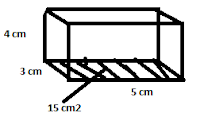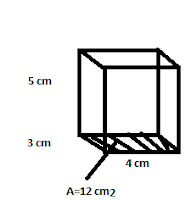2.

5. a)
Determine the volume of each right rectangular prism.

LxWxH LxWxH
3x4=12cm3 3x4=12 cm3
12x5=60 cm3 12x5=60cm3

8. Nina uses 15 centimeter cubes to make the base of the rectangular prism. Determine the volume if the prism has a total of 5 layers of cubes. Show your thinking.

If there are 5 layers of 15 cm cubes you times 15x5 which equals 75 cm3
5x15=75 cm3

10. A water through is in a shape of a right triangular prism with base area of 1250 cm2 and a height of 100 cm. How much water can be put in there before it overflows?

1250x100=125 000 cm3

Here is 1 question from 7.3 on page 265-267

4. Determine the volume of each cylinder.
a)h=23 cm
r=5 cm
d=2.r
d=5.2
d=10 cm
C=Pi.d
C=3.14x10
C=31.4
v=1805.5 cm3

b)r=14 cm
h=12 cm
d=r.2
d=14.2
d=28 cm
C=pi.d
C=3.14.28
C=87.92
V=7385.28 cm3

c)r=0.5 m
h=1.5
d=r.2
d=0.5.2
d=1 m
C=pi.d
C=3.14.1
C=3.14
V=1.177

9. The Canadarm has a cylinder called a capture envelope that is used to catch objects floating in space. It is 20.3 cm in diameter and 10 cm deep. What is the maximum volume it can hold?

7.4 Page 275
18. The volume of the triangular prism shown is 48 cm3. What is the volume of the missing measurement.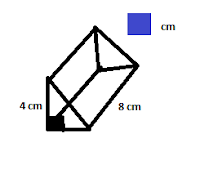5 cm3

### Karen's Volume Scribepost

SKY 248. What is the volume of the right cylinder?Pages 250-253 #'s 1, 4 and 7
1. Evan calculated the volume of a right cylinder. Charlotte calculated the volume of a right rectangular prism. Did either of them make an error in their solutions? Explain how you know.4. What is the volume of each right prism?
a) area of base = 12cm², height = 8cm3
b) area of base = 18cm², height = 4cm3
c) height = 9cm3, area of base = 14cm²

7. What is the height of each of the following right rectangular prisms?
a) volume = 32cm3, area of base = 8cm²
b) volume = 35cm3, area of base = 5cm²
c) area of base = 8cm², volume = 36cm3

Here is a video showing how to find the volume of a cylinder.
Here is a video showing how to find the volume of a rectangular prism.
*Sorry it's so messy, I can't fix it and I
'm too lazy to do it all over again.

Cylinder Volume and Volume ProblemsJumbo Popcorn:
r = d/2
r = 20/2
r = 10cm^2

areaofthebase x height
v= π*r*r*h
v= (3.14 x 10 x 10) x 40
v= 12 560cm^3

Popcorn Lover's:
r = d/2
r = 30/2
r = 15cm^2
areaofthebase x height
v = π*r*r*h
v = (3.14 x 15 x 15) x 20
v = 14 130cm^3
Martha should get the "Popcorn Lover's" if she wants more popcorn because the container is bigger by 1 570.r=d/2
r=1/2
r=0.5m^2
areaofthebase x height
v=π*r*r*h
v=(3.14*0.5*0.5)*10
v=7.85m^3

r=d/2
r=0.8/2
r=0.4m^2
areaofthebase x height
v=π*r*r*h
v=(3.14*0.4*0.4)*10
v=5.024m^3

7.85m^3*5.024m^3 = 2.862m^3

The volume required to make a concrete culvert is 2.862^3.

## Monday, February 14, 2011

### Glenn's Scribe Post Volume

Homework;
Pages 78-79 in the Home Work Book

2.4.a)400 cm3
b)339 cm3

c)960 cm3

6.a) Both of the rectangular prism has the volume of 200 cm3
b) Both of the rectangular prism has the volume of 10.5 m3

Sorry for not having a lot pictures on my blog because it was really hard to make 3D objects on paint.

Chapter 7 Section 7.3 Pages 246 to 253d/2=r 20/2=10
(2πrr)+(2rπh)
(2x3.14x10x10)+(2x10x3.14x40)
628+2512
3140 cm^

Chapter 7 Section 7.4 Pages 268 to 275

v= 280 cm^
then ..
v= 280x64
v= 17,920 cm^

### Tyler's Volume Post

Volume

Linear measurement is measuring a line with a ruler.

There are different units of measurement and some examples are centimeters, milimeters, and meters.

Area measurement is measuring an area with square units.

Volume measurement is measuring an area in cubic units.

If your measuring volume and it is a liquid, remember that 1cm3=1ml

The definition of volume- The amount of space an object occupies.

The formula for finding volume is area of base x height

The base is any face that shows the named face of the prism.

The height is the perpendicular distance between the two bases of a prism or cylinder.

Rectangular Prism

l x w =a
5 x 6
30cm2=a
30cm2 x 10cm = a
V= 300cm3

Triangular Prism

Area of base x height
b x h x h

-----------

2

6 x 10

-------- =30cm2
2

30cm2 x 5cm= 150cm3

r= d\2 area of base x height
r=10\2 pi•r2= a
r=5cm 3.14•25=a
78.5cm2=a
78.5cm2•10cm=785cm3

A rectangular prism has a length of 12cm, a width of 10cm, and a height of 15cm. What is the volume?